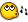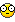## Recommended Posts

```;=======================================================
; Function: _AreaUnderCurve
;
; Uses Trapezoidal rule to give move accurate results
; Action: Performs an evaluated calculus integral over
;         a given range on a single variable expression
;         Read NOTES below before using
;
; \$expr: String - Correctly formatted math expression
; \$var: String - Independent variable
; \$lower_limit: Float - starting location
; \$upper_limit: Float - ending location
; \$numIntvs: Integer - Number of trapezoids under curve
;                    - Higher number = more accurate
; \$Places: Integer - Number of places to round result
; Returns: Area in float
;
; NOTE: upper_limit must be greator than lower_limit
; NOTE: Does not take into account integration x-intercepts
;       Therefore must integrate on those intercepts 1 by 1
; NOTE: A higher \$numIntvls is more accurate but too high will
;       take longer to calculate
;
; Ex 1) Calculate Pi
;    4*_AreaUnderCurve("Sqrt(1-((x)^2))","x",0,1,10000)
; Ex 2) Quadatic polynomial 2(x)^2 + 7x + 3
;   _AreaUnderCurve("2*(x)^2 + 7*x + 3","x",0,1,10000)
; Ex 3) Logorithm
;   _AreaUnderCurve("Log(x)","x",0,1,10000)
;=======================================================

Func _AreaUnderCurve(\$expr,\$var,\$lower_limit,\$upper_limit,\$numIntvs,\$Places = 4)
If Not StringInStr(\$expr,\$var) Then
ElseIf Not IsInt(\$numIntvs) Or \$numIntvs < 0 Then
return "Interval is < 0 or not an integer"
EndIf
Local \$dx = (\$upper_limit-\$lower_limit)/\$numIntvs
Local \$area, \$x, \$y = 0
If \$upper_limit < \$lower_limit Then
return "upper limit must be greater than lower limit"
ElseIf \$lower_limit >= 0 Or \$Upper_Limit <= 0 Then
\$y = Execute(StringReplace(\$expr,\$lower_limit,"\$x"))
For \$i = 1 To \$numIntvs - 1
\$x = (\$i*\$dx)
\$y += 2*Execute(StringReplace(\$expr,\$var,"\$x"))
Next
\$y += Execute(StringReplace(\$expr,\$upper_limit,"\$x"))
return Round(Abs(\$dx*\$y)/2,\$Places)
ElseIf \$lower_limit < 0 And \$upper_limit >= 0 Then
Local \$y_Neg, \$y_Pos = 0
\$y_Neg = Execute(StringReplace(\$expr,0,"\$x"))
For \$i = 1 To \$numIntvs - 1
\$x = (\$i*((Abs(\$lower_limit))/\$numIntvs))
\$y_Neg += 2*Execute(StringReplace(\$expr,\$var,"\$x"))
Next
\$y_Neg += Execute(StringReplace(\$expr,\$lower_limit,"\$x"))
\$y_Pos = Execute(StringReplace(\$expr,0,"\$x"))
For \$i = 1 To \$numIntvs - 1
\$x = (\$i*((\$upper_limit)/\$numIntvs))
\$y_Pos += 2*Execute(StringReplace(\$expr,\$var,"\$x"))
Next
\$y_Pos = Execute(StringReplace(\$expr,\$upper_limit,"\$x"))
return Round(Abs((\$y_Pos+\$y_Neg)*\$dx)/2,\$Places)
EndIf
EndFunc ;_AreaUnderCurve()==>```

Had to write this script for one of my courses in college.

It performs an integration of an expression over a specified interval and returns

the area under the curve.

Calculate Pi: _AreaUnderCurve("4*Sqrt(1-((x)^2))","x",0,1,10000)

Polynomial: _AreaUnderCurve("2*(x)^2 + 7*x + 3","x",0,1,10000)

Logorithm: _AreaUnderCurve("Log(x)","x",0,1,10000)

A* (A-star) Searching Algorithm - A.I. Artificial Intelligence bot path finding

##### Share on other sites

Good job. I really had no idea how to do this programming-wise..##### Share on other sites

Good job. I really had no idea how to do this programming-wise..just use the definition of an integral. like he did##### Share on other sites

just use the definition of an integral. like he didI would never add values when you take tiny steps in a formula, lol. I'd just calculate it the way everyone does it. Edited by Manadar
##### Share on other sites

I would never add values when you take tiny steps in a formula, lol. I'd just calculate it the way everyone does it.

Why not? rofl

A* (A-star) Searching Algorithm - A.I. Artificial Intelligence bot path finding

##### Share on other sites

Why not? rofl

:|

Ok... Integral for f(x) = x between 0 and 1 .. We all know this is 1. Now, what you do is this:

Result = 0

Take a tiny step.. Get the value of x there (0.00001)

Result = 0.00001

Take a tiny step.. Get the value of x there (0.00001)

Result = 0.00002

Take a tiny step.. Get the value of x there (0.00001)

Result = 0.00003

Take a tiny step.. Get the value of x there (0.00001)

Result = 0.00004

Take a tiny step.. Get the value of x there (0.00001)

Result = 0.00005

Take a tiny step.. Get the value of x there (0.00001)

Result = 0.00006

Take a tiny step.. Get the value of x there (0.00001)

Result = 0.00007

Take a tiny step.. Get the value of x there (0.00001)

Result = 0.00008

Take a tiny step.. Get the value of x there (0.00001)

Do I have to continue?##### Share on other sites

Yes I know I was joking. This function takes advantage of CPU power not integration rules as iin calculus. I just found programming this function fun.. Not the most efficient way to calculate PI though, to get PI accuratly to 5 decimal places it takes my PC 38 seconds lol. But still, neat concept..

A* (A-star) Searching Algorithm - A.I. Artificial Intelligence bot path finding

##### Share on other sites

actually the integral for f(x) = x between 0 and 1 is 0.5##### Share on other sites

actually the integral for f(x) = x between 0 and 1 is 0.5I see you been using the function. lol jkA* (A-star) Searching Algorithm - A.I. Artificial Intelligence bot path finding

##### Share on other sites

actually the integral for f(x) = x between 0 and 1 is 0.5Lol! I can't believe I had that wrong... :">

I know it is 0.5 ...##### Share on other sites

• 2 weeks later...

Is this useful? or do people not do this type of math?

A* (A-star) Searching Algorithm - A.I. Artificial Intelligence bot path finding

## Create an account

Register a new account

• ### Recently Browsing   0 members

×

• Wiki

• Back

• #### Beta

• Git
• FAQ
×
• Create New...